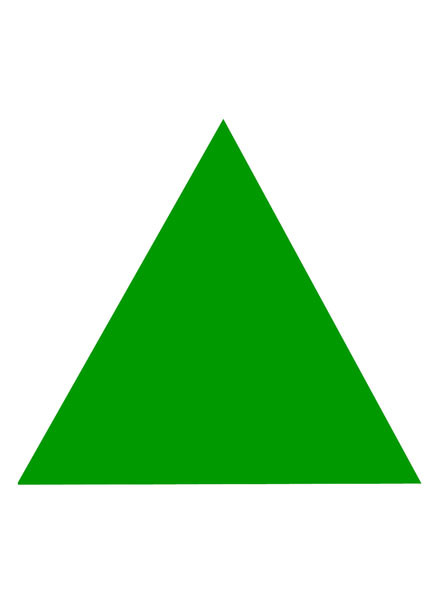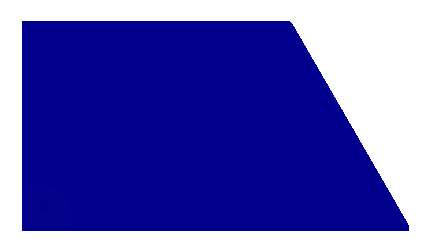# Figures

Learning to recognize geometric figures. Learning through play, multimedia tests to help recognize and classify figures at the preschool level.

Test timer:: 0:0:0

Points Sum: 1/1

Question timer: 0:0:0

Question: 1/1

Max points: 1

Go back

Average stars: 0

Average stars count: 0

Average points: 0

Average time: 00:01:52

Average time count: 79square

triangle

rectangle

rectangle

rectangle

rectangle

circle

trapeze

trapeze

trapeze

triangle

trapeze

square

square

square

circle

square

circle

rectangle

circle

trapeze

triangle

triangle

circle

triangle